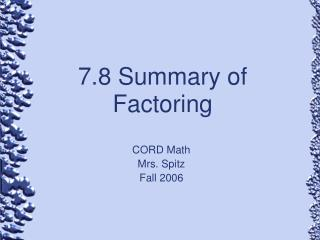# 7.8 Summary of Factoring - PowerPoint PPT PresentationDownload Presentation7.8 Summary of Factoring

7.8 Summary of FactoringDownload Presentation## 7.8 Summary of Factoring

- - - - - - - - - - - - - - - - - - - - - - - - - - - E N D - - - - - - - - - - - - - - - - - - - - - - - - - - -
##### Presentation Transcript

1. 7.8 Summary of Factoring CORD Math Mrs. Spitz Fall 2006

2. Objective: • Factor polynomials by applying various methods of factoring.

3. Assignment • Pg. 288 #5-50 all

4. Introduction • In this chapter, you have used various methods to factor different types of polynomials. The following chart on the next slide summarizes these methods and can help you decide when to use a specific method.

5. Summary Chart

6. Watch . . . • Whenever there is a GCF other than 1, always factor it out first. Then check the appropriate factoring methods in the order shown in the table. Use these methods to factor until all of the factors are prime.

7. Ex. 1: Factor 3x2 - 27 • First check for a GCF. Then since there are two terms, check for the difference of squares. • Thus 3x2 – 27 is completely factored as 3(x +3)(x – 3) 3 is the GCF x2 – 9 is the difference of squares since x  x = x2 and 3  3 = 9

8. Ex. 2: Factor 9y2 – 58y + 49 • The polynomial has three terms. So check for the following: • GCF – The GCF is 1 • Perfect square trinomial – Although 9y2 = (3y)2 and 49 = (7)2, 58y ≠ 2(3y)(7). • Trinomial w/ two binomial factors: Are there two numbers whose product is 9  49 or 441 and whose sum is -58?

9. Ex. 2: Factor 9y2 – 58y + 49 • You must find two numbers whose product is 9 · 49 or 441 and whose sum is -58.

10. Ex. 2: Factor 9y2 – 58y + 49 • Trinomial w/ two binomial factors: Are there two numbers whose product is 9  49 or 441 and whose sum is -58? • YES, the product of -9 and -49 is 441 and their sum is -58. -58 -49 -9 441 (9y – 49)(y – 1) Check this by using FOIL.

11. Ex. 3 – Factor by grouping Pull GCF of 2mn Group two commons together Pull m2 out of first group and -4 out of 2nd group. Recognize Difference of squares Factor remaining and simplify. You can check this by multiplying the factors.

12. Chalkboard examples #1 • Factor 5ax2 – 45a

13. Chalkboard examples #2 • Factor 32x3 – 50x

14. Factor x4 + x3 – 12x2 GCF of x2 Factors of -12 that subtract to give you 1. Chalkboard examples #3

15. Factor 12a3 – 16a2 – 16a GCF of x2 Factors of -12 that subtract to give you -4. Chalkboard examples #4

16. CB ex. 4: Factor 3a2 – 4a – 4 • Trinomial w/ two binomial factors: Are there two numbers whose product is 3  -4 or -12 and whose sum is -4? • YES, the product of -6 and 2 is -12 and their sum is -4. -4 +2 -6 -12 (a - 2)(3a + 2) Check this by using FOIL.